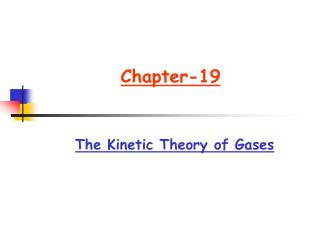DownloadDownload PresentationChapter-19

# Chapter-19

Download Presentation## Chapter-19

- - - - - - - - - - - - - - - - - - - - - - - - - - - E N D - - - - - - - - - - - - - - - - - - - - - - - - - - -
##### Presentation Transcript

1. Chapter-19 The Kinetic Theory of Gases

2. Chapter-19 The Kinetic Theory of Gases • Topics to be covered: • Ideal gas law • Internal energy of an ideal gas • Distribution of speeds among the atoms in a gas • Specific heat under constant volume • Specific heat under constant volume. • Adiabatic expansion of an ideal gas

3. Ch 19-2, 3 Avogadro Number • M= m NA ; N= nNA; Msamp=n M NA • Ideal gas law: An ideal gas obey the law pV=nRT where R=8.31 J/mol.K • Boltzman constant k k = R/NA • pV=nRT=NkT • Kinetic Theory of gases: Relates motion of atoms to the volume, pressure and temperature. • Mole: one mole is number of atoms in a 12 g sample of carbon-12 • Avogadro Number: one mole contains Avogadro number NA of atoms NA = 6.02 x 1023 atoms/mol • n= number of moles • N= number of molecules • M= Molar mass of a substance • Msamp= mass of a sample then

4. Ch 19-3 Ideal Gas and work done by the ideal gas • Work done by an ideal gas at constant Temperature (Isothermal expansion ): An ideal gas is allowed to expand from initial state pi,Vi to pf,Vf at constant T, the work W is: W=VfVipdV=VfVi (nRT/V)dV= W=nRTln(Vf/Vi) • Work done by an ideal gas at constant volume: W=VfVipdV=0 and Eint=Q • Work done by an ideal gas at constant pressure W=p(Vf-Vi)=pV=nRT

5. Ch 19-4,5 Pressure, Temperature, RMS Speed and Translational Kinetic Energy • Pressure p relation to root-mean -square speed vrms and temperature T • P=(nM vrms 2)/3V but pV=nRT then • Vrms =  [(3RT)/M] • Translational Kinetic Energy K: Average translational kinetic energy of a molecule Kavg=(mv2/2)avg=m(vrms2)/2 • Kavg=m(vrms2)/2=(m/2)[3RT/M] =(3/2)(m/M)RT=(3/2)(R/NA)T Kavg=(3/2)(R/NA)T=(3/2)kT

6. Ch 19-8 The Molar Specific Heats of an Ideal Gas • Internal Energy Eint: Ideal gas is monatomic and its Eint is sum of translational kinetic energies of its atom. For a sample containing n moles, its internal energy Eint: Eint=nNAKavg=nNA(3/2)kT= (3/2)n(NAk)T Eint= (3/2)nRT • Molar Specific Heat at Constant Volume For an ideal gas process at constant volume pi,Ti increases to pf,Tf and heatabsorbed QV=nCVT and W=0. Then Eint= (3/2)nRT= Q=nCVT CV= 3R/2 Where CV is molar specific heat at constant volume

7. Ch 19-8 The Molar Specific Heats of an Ideal Gas • Cp :Molar Specific Heat at Constant Pressure: For an ideal gas process at constant pressure Vi,Ti increases to Vf,Tf and heatabsorbed QP=nCpTand W=pV= nR T. Then Eint= (3/2)nRT= Q-W =nCpT- nRT. Cp= 3R/2+R=5R/2 Where Cp is molar specific heat at constant pressure • Cp= CV + R; specic heat ration = Cp/ CV For monatomic gas Cp= 5R/2 ; CV= 3R/2 and = Cp/ CV = 5/3

8. Ch 19-11 The Adiabatic Expansion of an Ideal Gas • Adiabatic process:In anadiabatic expansion of an ideal gas no heat enters or leaves the system i.e. Q=0 • P, V and T are related to the initial and final states with the following relations: PiVi= PfVf TiVi-1 = TfVf-1 • Also T/( -1) V =constant then piTi(-1)/ = pfTf(-1)/

9. Ch 19-11 The Adiabatic Expansion of an Ideal Gas Free Expansion • Free Expansion of an ideal gas- • An ideal gas expands in an adiabatic process such that no work is done on or by the gas and no change in the internal energy of the system i.e. Ti=Tf • Also in this adiabatic process since ( pV=nRT), piVi=pfVf ( notPiVi= PfVf)

10. Suggested problemsChapter 19